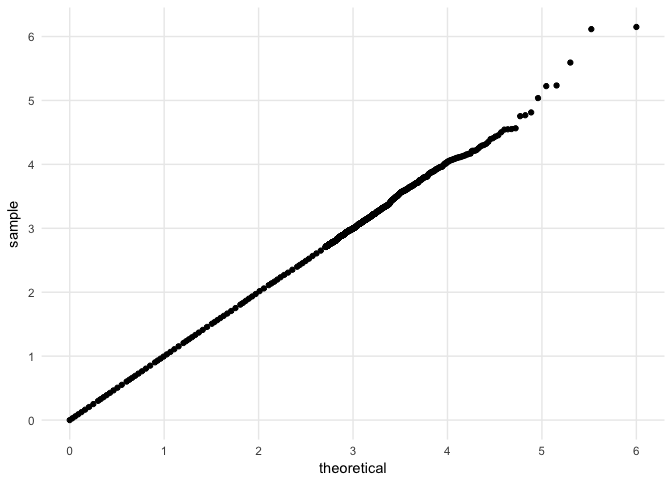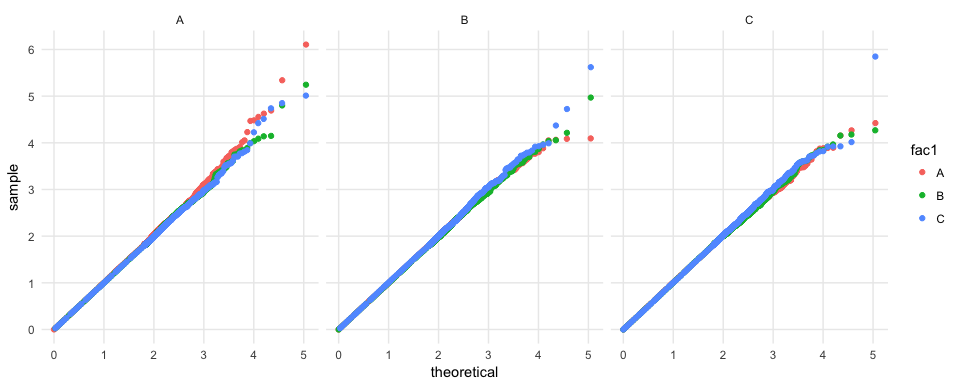# Compare Big Datasets to the Uniform Distribution

A quantile-quantile plot can be used to compare a sample of p-values to the uniform distribution. But when the dataset is big (i.e. > 1e4 p-values), plotting the quantile-quantile plot can be slow. geom_QQ uses all the data to calculate the quantiles, but thins it out in a way that focuses on points near zero before plotting to speed up plotting and decrease file size, when vector graphics are stored.

ggbigQQ extends `ggplot2` to allow the user to make a quantile-quantile plot with a big dataset. Specifically, `geom_big_qq` uses all the data provided to calculate quantiles, but drops points that would overplot before plotting. <!-- There's no use in having ten thousand points in a plot to define a line -- we can't even see most of them! --> In this way, the resultant figure maintains all the accuracy of a Q-Q plot made with a large dataset, but renders as fast as one from a smaller dataset and, when stored as a vector graphic, has the file size of a Q-Q plot from a smaller dataset.

## Examples

Here's an example where `geom_qq` takes 14 seconds to render the plot on my intel i5 and `geom_big_qq` takes 1 second to produce the same plot.This `geom` works with other aesthetics, too.# Reference manual

install.packages("ggQQunif")

0.1.5 by Robert Corty, 3 years ago

Browse source code at https://github.com/cran/ggQQunif

Authors: Robert Corty [aut, cre]

Documentation:   PDF Manual